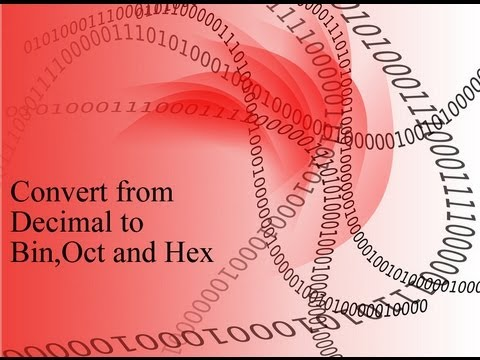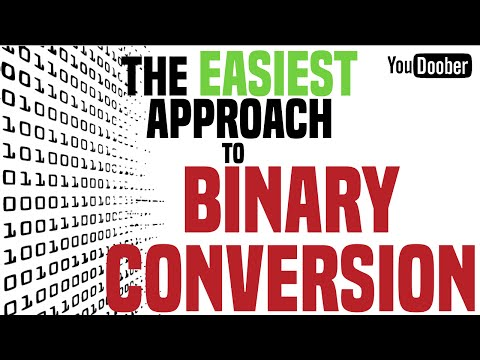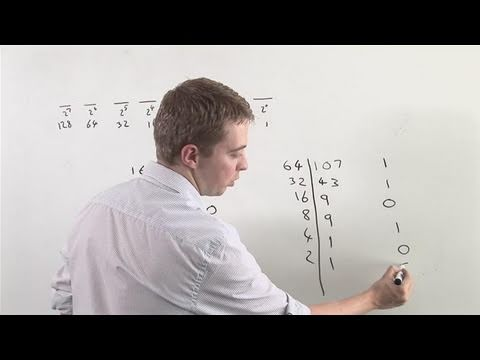## Decimal and Binary Number

Subject: Computer Science

Find Your Query

#### Overview

The number of base or radix ten is called decimal numbers. Similarly, Binary Number is a number of two base numbers.This note provides an information about Decimal and Binary Numbers.

#### Number System

History of number starts from primitive age of human being. The development of number system has integrated with the development of human beings. In primitive age, people used to count stones and pebbles. Hindu philosophers have developed the number system called decimal numbers. From the number various other numbers are derived and developed. These numbers are binary numbers, octal numbers, hexadecimal numbers etc.

#### Decimal (Denary) Numbers

The number of base or radix ten is called decimal numbers. It is first number system in which all the ancient and modern mathematical calculation is done. Other number system is derived from this number. It is generated with the combination of 0, 1, 2, 3, 4, 5, 6, 7, 8, 9. We can represent these numbers with suffix two. Eg (9810)10

The following chart displays relations among several number systems derived from the decimal number.

 System Base 0 1 2 3 4 5 6 7 8 9 10 11 12 13 14 15 Hexadecimal 16 0 1 2 3 4 5 6 7 8 9 A B C D E F Decimal 10 0 1 2 3 4 5 6 7 8 9 Octal 8 0 1 2 3 4 5 6 7 Binary 2 0 1

#### Binary Number

Binary Number is a number of two base numbers. It is represented by 1 and 0. 1 or 0 is called Binary digits.

We can generate this number with the combination of 0 and 1. It is represented with suffix two. Eg (10101)2.

Following table shows some decimal number and their equivalent binary numbers.

 Decimal Binary 0 0 1 1 2 10 3 11 4 100 5 101 6 110 7 111 8 1000 9 1001

#### Conversion of Binary to Decimal and vice versa

We multiply each binary digit by its weighted position, and add each of the weighted value together.

Weighted value:

 25 24 23 22 21 20 32 16 8 4 2 1To convert decimal number to binary number,repeated division by two is needed.

#### Convert following into binary numbers:

(45)10= (101101)2

 2 45 1 2 22 0 2 11 1 2 5 1 2 2 0 1

=(101101)2

(225)10= (10111001)2

 2 225 1 2 112 0 2 56 0 2 23 1 2 11 1 2 5 1 2 2 0 1

= (10111001)2

##### Things to remember
• The arrangement of number in a computer system is known as computer number system.
• There are different types of computer number system.They are binary number system( have base 2), octal number system(have base 8), decimal number system(have base 10 ) and hexadecimal number system(have base 16).
• Binary Number is a number of two base numbers. It is represented by 1 and 0.
• The number of base or radix ten is called decimal numbers.
• It includes every relationship which established among the people.
• There can be more than one community in a society. Community smaller than society.
• It is a network of social relationships which cannot see or touched.
• common interests and common objectives are not necessary for society.
##### Videos for Decimal and Binary Number##### Convert a decimal number to binary, octal, and hexadecimal##### How To Convert Binary To Decimal Tutorial (The Easy Way)##### Questions and Answers

A number system act as the symbols used to express quantities on the basis for counting, comparing amounts, performing calculations, and representing value. The different number system use different digits or symbols to represent numbers. The number of digits used in a number system is known as base of the number system. The different number systems have different base number. The different types of number system are: -

• Decimal Number System
• Binary Number System
• Octal Number System
• Hexadecimal Number System

A number system that uses ten different digits to represent different values is known as decimal number system. The base of decimal number system is 10 because it consist ten digits from 0 to 9. Decimal number can be expressed by using powers of 10.

A positive decimal integer can be converted to binary through successive division by 2 till the quotient becomes zero and sequential collection of remainder on last come first basis (i.e. bottom to top).

Solution:

 Remainder 2 349 -1 2 174 -0 2 87 -1 2 43 -1 2 21 -1 2 10 -0 2 5 -1 2 2 -0 2 1 -1 0

Hence, (349)10 = (101011101)2

A positive decimal integer can be converted to octal through successive division by 8 till the quotient becomes zero and sequential collection of remainder on last come first basis (i.e. bottom to top).

Solution:

 Remainder 8 427 -3 8 53 -5 8 6 -6 0

Hence, (427)10 = (653)2

A positive decimal integer can be converted to hexadecimal through successive division by 16 till the quotient becomes zero and sequential collection of remainder on last come first basis (i.e. bottom to top). But the remainder 10 or above is represented by the capital letters from A to F respectively.

Solution:

 Remainder 16 1495 -7 16 93 -13 (D) 16 5 -5 0

Hence, (1495)10 = (5D7)16

A number system that uses two different digits to represent different values is known as binary number system. The base of binary number system is 2 because it consist two digits 0 and 1. Each digit of the binary number system is called Binary Digit (BIT). The binary number system is used in the computer.

The decimal equivalent of a binary number is the sum of the digits multiplied by 2 with their corresponding weights.

Solution:

8 7 6 5 4 3 2 1 0 (weight)

Binary number: 1 0 1 0 1 1 1 0 1

Decimal equivalent: 1×28 + 0×27 + 1×26 + 0×25 + 1×24 + 1×23 + 1×22 + 0×21 + 1×20

=1×256 + 0×128 + 1×64 + 0×32 + 1×16 + 1×8 + 1×4 + 0×2 + 1×1

=256 + 0 + 64 + 0 + 16 + 8 + 4 + 0 + 1

= (349)10

Hence, (1010111101)2 = (349)10

Octal digit is represented in 3 bits. So, a binary number is converted to its octal equivalent by grouping their successive 3 bits of the binary number starting from the least significant bit (right-most digit) and then replacing each 3 bit group by its octal equivalent.

Binary Table

 Octal Binary 0 000 1 001 2 010 3 011 4 100 5 101 6 110 7 111

Solution:

Binary Number: 1 0 1 1 1 0 1 1 1 1 0

Paired Binary Digits: 10 111 011 110

Octal Equivalent : 2 7 3 6 (From Binary Table)

Hence, (10111011110)2 = (2736)8

Hexadecimal digit is represented in 4 bits. So, a binary number is converted to its hexadecimal equivalent by grouping together successively 4 bits of the binary number starting from the least significant bit (right-most digit) and then replacing each bit-group by its hexadecimal equivalent.

Binary Table

 Hexadecimal Binary 0 0000 1 0001 2 0010 3 0011 4 0100 5 0101 6 0110 7 0111 8 1000 9 1001 A 1010 B 1011 C 1101 D 1110 E 1111

Solution:

Binary Number: 1 0 1 1 0 1 1 0 1

Paired Binary Number: 1 0110 1101

Hexadecimal Equivalent: 1 6 D

Hence, (101101101)2 = (16D)16

##### Quiz

© 2019-20 Kullabs. All Rights Reserved.